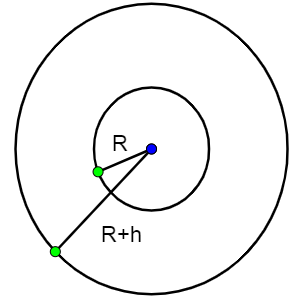QuestionAnswers

# A satellite is launched in the equatorial plane in such a way that it can transmit signals upto $60 ^{\circ}$ latitude on the earth. Then the angular velocity of the satellite is:A. $\dfrac{GM}{8R^3}$B. $\dfrac{GM}{2R^3}$C. $\dfrac{GM}{4R^3}$D. $\sqrt{\dfrac{3\sqrt{3}GM}{8R^3}}$Verified
129k+ views
Hint: The question is from the chapter ‘Gravitation’ and can be solved by using the formula of the time period of the satellite in an orbit. Angular velocity of a body is inversely related to its time period.
Formula used:
Formula for the time period of a revolving object:
$T=2\pi \sqrt{\dfrac{r^{3}}{GM}}$
The angular velocity is related to time period as:
$\omega=\dfrac{2\pi}{T}$

Given:
The range of the satellite: $60 ^{\circ}$ latitude of earth.
The orbital time period of a satellite orbiting in earth's orbit, at a height of r=R+h is given as:
$T=2\pi \sqrt{\dfrac{r^{3}}{GM}}$The angular velocity is given as:
$\omega=\dfrac{2\pi}{T}$
We require finding the height r of the satellite.
If we consider the fact that $60 ^{\circ}$ latitude of earth is nothing but $R\tan 60 ^{\circ}$. This is the range, with R being the radius of earth. The range satellite, for the point where it is at a height h above the equatorial plane :
Range= $\sqrt{(R+h)^{2}-h^2}$
Thus, in our case
$R\dfrac{1}{\sqrt{3}}=$$\sqrt{(R+h)^{2}-h^2}$
$\dfrac{R^2}{3}=2Rh+h^2$

By solving, this quadratic equation, we get:
$h=-R \pm \dfrac{2 R}{\sqrt{3}}$
Thus, using the formula for time period now,
r=R+h;
$r=\dfrac{2R}{\sqrt{3}}$
$T=2\pi \sqrt{\dfrac{(2 R / \sqrt{3})^{3}}{GM}}$

Thus the angular velocity is obtained as
$\omega=\dfrac{2 \pi}{2 \pi \sqrt{\dfrac{(2 R / \sqrt{3})^{3}}{GM}}}$
$\sqrt{\dfrac{3\sqrt{3}GM}{8R^3}}$

So, the correct answer is “Option D”.